# Theoretical review of ADC methods¶

The polarisation propagator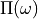is a quantity from many-body perturbation theory [Fet71]. Its relationship to electronically excited states spectra can be understood from the fact that its poles are located exactly at the vertical excitation energies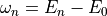[Fet71][Sch82]. Here,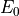is the energy of the ground state of the exact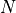-electron Hamiltonian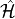, and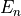is the energy corresponding to excited state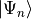. The structure ofclose to these poles depends both on the ground state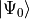and excited state, such that, e.g., transition properties [Sch82][Sch18] may be extracted fromas well.

Taking this as a starting point, the algebraic-diagrammatic construction scheme for the polarisation propagator (ADC) examines an alternative representation of the polarisation propagator [Sch82], the so-called intermediate-state representation (ISR). In this formalism, a set of creation and annihilation operators is applied to the exact ground state and the resulting precursor states are orthogonalised block-wise according to excitation class [Sch91]. This procedure yields the so-called intermediate states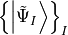, which are then employed to represent the polarisation propagator. A careful inspection of the resulting expression forand its poles allows to relate the intermediate states (IS) to the excited statesof the Hamiltonian [Sch91] by a unitary transformation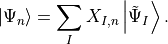The expansion coefficients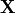satisfy a Hermitian eigenvalue problem [Sch91]

(1)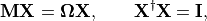where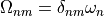is the diagonal matrix of excitation energies and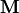is the so-called ADC matrix. Its elements are directly accessible by representing the shifted Hamiltonian using IS, namely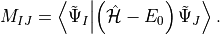From the ADC eigenvectorsin the IS basis, one may compute the density matrix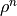for an excited state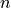or the transition density matrices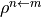between state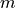and, in the molecular orbital (MO) basis [ST04][WRH+14]. Contracting these densities with the MO representation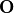of a one-particle operator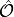allow to compute arbitrary state properties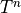or transition properties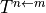through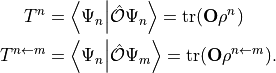In this way, e.g., the MO representation of the dipole operator may be contracted withto obtain the transition dipole moment between statesandand from this the oscillator strength. Linear and non-linear molecular response properties, e.g., static polarizabilities or two-photon absorption cross-sections, are also accessible via this framework [TKWS06][KRW+12][FRDN17].

As described so far, the above formalism builds the IS basis on top of the exact-electron ground state and is thus exact as well. For practical calculations, however, the ADC scheme is not applied to the exact ground state, but to a Møller-Plesset ground state at orderof perturbation theory. The resulting ADC method is named ADC() and is by construction consistent with an MP() ground state. Detailed derivations and the resulting expressions for the ADC matrixas well as the aforementioned densitiesandfor various orders can be found in the literature [Sch82][Sch91][WRH+14][DW14][Sch18] and will not be discussed here.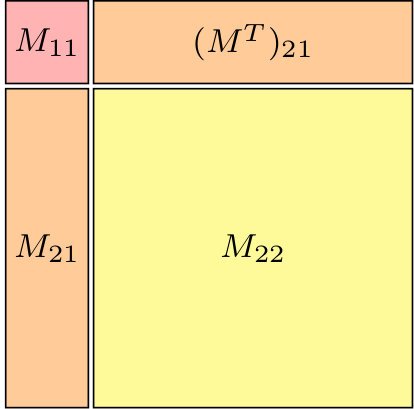Fig 1a. Schematic ADC matrix¶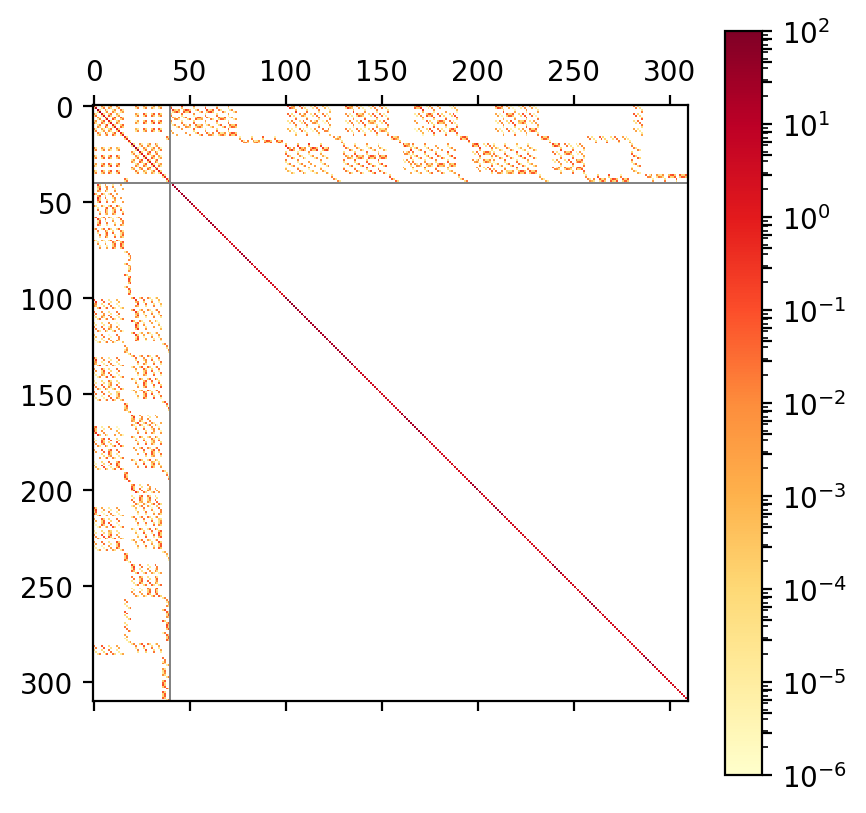Fig 1b. ADC(2) matrix of STO-3G water¶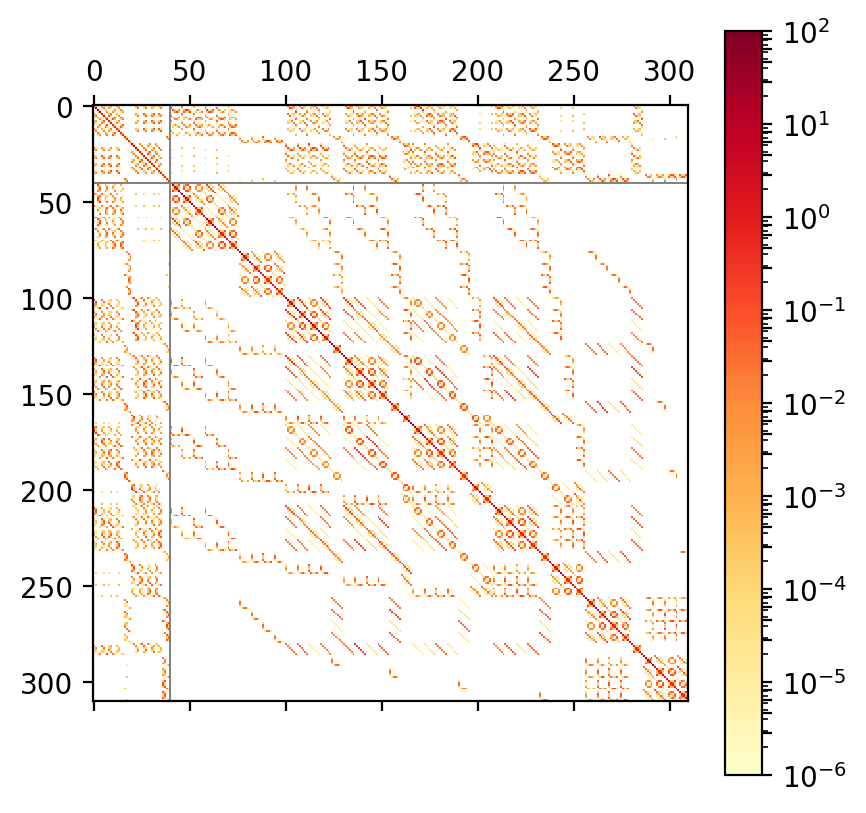Fig 1c. ADC(3) matrix of STO-3G water¶

As a result of the construction of ADC() as excitations on top of an MP() ground state, the matrixexhibits a block structure, shown in Figure 1a. In this the singles block is denoted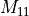, the doubles block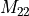and the coupling block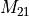. One may construct perturbation expansions for the individual blocks as well. For example in ADC(2) the lower-rightblock is only present in zeroth order. In ADC(3) on the other hand this block is present at first order, which makes it consistent with an MP(3) ground state. In contrast, ADC(2)-x is an emph{ad hoc} modification of ADC(2), where only the doubles-doubles block is treated first order like in ADC(3), but the remaining blocks remain at the same order as in ADC(2) [DW14].

On top of this block structure the individual blocks are sparse as well, see Figure 1b and c. This sparsity is a direct consequence of the selection rules obtained from spin and permutational symmetry in the tensor contractions required for computing. To exploit this sparsity when diagonalising the matrix (1), adcc follows the conventional approach [DW14][WRH+14] to use contraction-based, iterative eigensolvers, such as the Jacobi-Davidson [Dav75]. Furthermore, all tensor operations in the required ADC matrix-vector products are performed on block-sparse tensors. For an optimal performance the spin and permutational symmetry of the ADC equations need to be taken into account when setting up the block tiling along the tensor axes. In this setting the computational scaling of ADC(2) is given as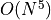whereis the number of orbitals, whereas ADC(2)-x and ADC(3) scale as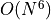. This procedure additionally ensures the numerical stability of the eigensolver with respect to the excitation manifold. That is to say, that (for restricted references) spin-pure guess vectors always lead to eigenvectorsfrom the same manifold, such that the excitation manifold to probe can be reliably selected via the guesses without employing a spin-adapted basis. [DW14]

One important modifications of the ADC scheme as discussed above is the core-valence separation (CVS) [CDS80][TMG+00][WWD14b][WWD14a][WHWD15]. In this approximate ADC treatment targeting core-excited states, the strong localisation of the core electrons and the weak coupling between core-excited and valence-excited states is exploited to decouple and discard the valence excitations from the ADC matrix. This lowers the number of the actively treated orbitals and thus the computational demand for solving the ADC eigenproblem (1). The validity of this approximation has been analysed in the literature and is backed up by computational studies comparing with experiment [ND18][FD19]. With this, ADC can be used for considering core-excited states, and subsequent studies have also established the ability of calculating non-resonant X-ray emission spectra [FD19] and resonant inelastic X-ray scattering [RDN17]. Other variants of ADC include spin-flip [LWD15], where a modified Davidson guess allows to treat processes of simultaneous excitation and spin-flip, tackling few-reference problems in an elegant and consistent way [LRD16][LTMartinezD17]. Similar to other CI-like methods the range of orbitals which are considered for building the intermediate states may also be artificially truncated. For example, when considering valence-excitations, excitations from the core orbitals may be dropped leading to a frozen-core (FC) approximation. Similarly, high-energy virtual orbitals may be left unpopulated, leading to a frozen-virtual (FV) or restricted-virtual approximation [YD17].